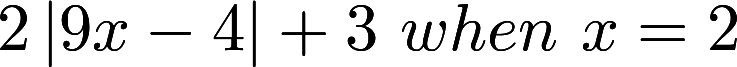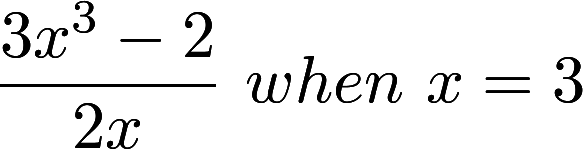• If you are citizen of an European Union member nation, you may not use this service unless you are at least 16 years old.

• Work with all your cloud files (Drive, Dropbox, and Slack and Gmail attachments) and documents (Google Docs, Sheets, and Notion) in one place. Try Dokkio (from the makers of PBworks) for free. Now available on the web, Mac, Windows, and as a Chrome extension!

View

# A1-A-3-4

last edited by 3 years, 6 months ago

A1.A.3.4 Evaluate linear, absolute value, rational, and radical expressions. Include applying a nonstandard operation such as.

In a Nutshell

Students will simplify expressions which include linear, absolute value, rational and radical using the order of operations.  Students will also use similar methods to simplify nonstandard expressions.

## Teacher Actions

• Students will develop accurate and appropriate procedural fluency as they evaluate a variety of algebraic expressions, including absolute value, radicals, or rationals,  for given values of the variables..

• Students will develop a deep and flexible conceptual understanding by examining different algebraic properties to determine where they should be used and also apply the understanding of these properties to nonstandard operations.

• Work alongside students to establish mathematical goals which focus learning on the students’ explanations and processes, including finding equivalent expressions.

• Facilitate meaningful mathematical discourse to provide students with opportunities to find the mistake in order of operations or see that different order produces different results.

• Pose purposeful questions that encourage students to focus on patterns within and among expressions, especially which features of an expression are changing and which are staying the same?

## Misconceptions

• Evaluate linear expressions for the given values using the order of operations.

• Evaluate 2x+3y when x=3 and y=2

2(3) + 3(2)

6+6

12

• Evaluate expressions involving absolute values using the order of operations and implied grouping symbols.

• Evaluate• Evaluate expressions involving rationals using the order of operations and implied grouping symbols.• Evaluate expressions involving radicals (square and cube roots) using the order of operations and implied grouping symbols.

• Evaluate expressions using nonstandard operations, also known as binomial operation.
• Example:• `(Order of Operations) is often used improperly and students believe multiplication should occur before division and addition should occur before subtraction when in reality multiplication and division are one step (from left to right) along with addition and subtraction.

• Do not recognize implied grouping symbols when evaluating expressions involving radicals and fractions

• Incorrectly evaluate the expressionand get the incorrect resultrather than the correct answer of 7.

• Not all calculators follow correct order of operations.

• Students sometimes mistakenly interchange the mathematical words "expression" and "equation”

• Students will raise the variable by the power and forget to raise the coefficient by the same power.  Ie (2x2)2=2x4

• Students will incorrectly add non-like terms.

• When evaluating x2 for a negative value, students will evaluate using their calculator without using parentheses and therefore will not have the correct sign. Ex: Evaluating x2 for x = -3 should result in 9, but entering -32 in a calculator will result in -9.

• When using absolute value bars, students will imply to either change the signs of values or the negative values instead of simplifying the expression inside the bars before taking the absolute value of an expression.

OKMath Framework Introduction

Algebra 1 Introduction